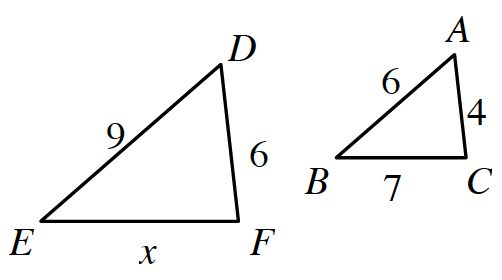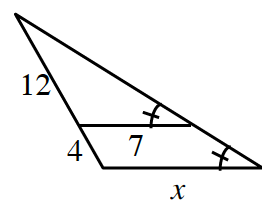### Home > CC3 > Chapter Ch10 > Lesson 10.1.5 > Problem10-69

10-69.

First decide if the triangles below are similar. Then, if they are similar, use the information in each diagram to solve for $x$.

1.If only two pair of corresponding sides have equal ratios, can you conclude that the triangles are similar?

1. Careful!The two triangles are similar (by $\text{AA}\sim$).

Draw two separate triangles, then write a proportion and solve for $x$.

$\frac{12}{7}=\frac{16}{x}$